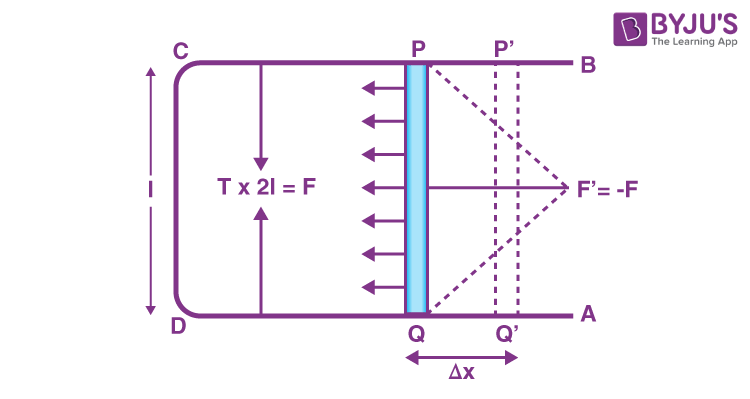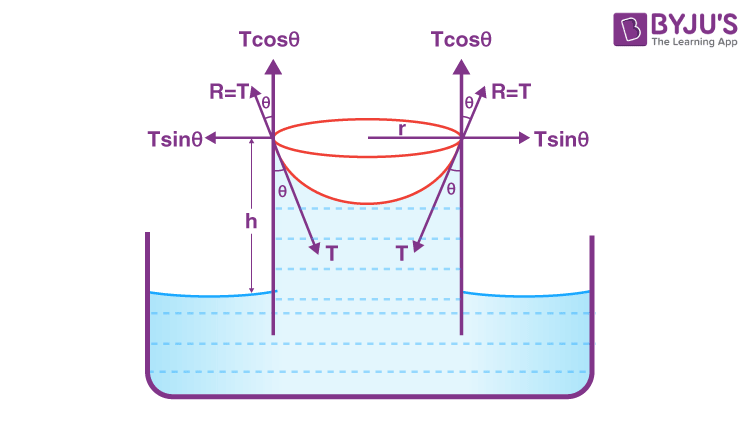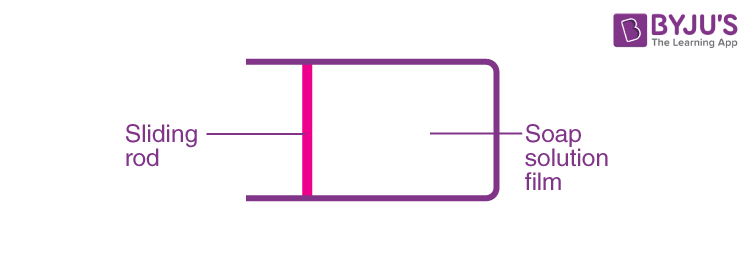Jet Set Go! All about Aeroplanes Jet Set Go! All about Aeroplanes

# Surface Tension Questions

Surface tension is the tendency of liquid surfaces at rest to shrink to the smallest possible surface area. Surface tension is what allows objects with a higher density than water, such as razor blades and insects (such as water striders), to float on the water’s surface without becoming submerged.

Surface tension at liquid-air interfaces is caused by the greater attraction of liquid molecules to each other (due to cohesion) than to air molecules (due to adhesion).

There are two main mechanisms at work. The first is an inward force on the surface molecules, which causes the liquid to constrict. The second force is a tangential force parallel to the liquid’s surface. This tangential force is commonly known as surface tension.

Definition: Surface tension is defined as the phenomenon that occurs when the surface of a liquid comes into contact with another phase (it can be a liquid as well). Liquids tend to have the smallest possible surface area. The liquid’s surface behaves like an elastic sheet.

“Surface tension is the tension of a liquid’s surface film caused by the bulk of the liquid’s attraction of the particles in the surface layer, which tends to minimise surface area.”

## Surface Tension Questions with Solutions

Q1. Surface tension may be defined as-

(a) The work done per unit area in increasing the surface area of a liquid under isothermal conditions.

(b) The work done per unit area in increasing the surface area of a liquid under adiabatic conditions.

(c) The work done per unit area in increasing the surface area of a liquid under both isothermal and adiabatic conditions.

(d) Free surface energy per unit volume.

Q2. Water does not spread over the surface of oil because-

(a) the surface tension of water is very high.

(b) the surface tension of water is very low.

(c) the viscosity of oil is high.

(d) the viscosity of water is high.

Correct Answer- (a) the surface tension of water is very high.

Explanation. Water has a higher surface tension than oil. As a result, when oil is poured over water, the higher surface tension of the water pulls the oil in all directions, causing it to spread on the water. Water, on the other hand, does not spread over oil because the surface tension of oil is lower than that of water, making it unable to pull water over it.

Q3. Small droplets of a liquid are usually more spherical in shape than larger drops of the same liquid because-

(a) Force of surface tension is equal and opposite to the force of gravity.

(b) Force of surface tension predominates the force of gravity.

(c) Force of gravity predominates the force of surface tension.

(d) Force of gravity and force of surface tension act in the same direction and are equal.

Explanation. Surface tension can withstand small gravitational forces in small drops, but in large drops, gravitational force dominates surface tension, causing the large drop to bulge more.

Q4. Which type of fluids will have zero surface tension?

(a) Real fluids.

(b) Ideal fluids.

(c) Both ideal and real fluids.

(d) No fluids have zero surface tension.

Explanation. In an ideal fluid, the intermolecular forces are assumed to be very less. Therefore, they have zero surface tension.

Q5.Water’s surface tension can be reduced by-

(a) Dissolving common salt in water.

(b) Lowering water temperature.

(d) All of the above

Explanation. Detergent weakens the hydrogen bond that holds water molecules together at the surface, lowering surface tension.

Q6. Insects move on the surface of the water without sinking due to-

(a) Dynamic lift.

(b) Viscosity of water.

(c) Surface tension of water.

(d) Buoyant force.

Correct Answer. (c) Surface tension of water

Explanation. Some insects can easily walk on water due to the weight of the insect and the structure of their feet, combined with the surface tension of the water.

Q7. Two soap bubbles of unequal sizes are blown at the ends of a capillary tube. Which one will grow at the expense of the other and what does it show?

Answer. The larger will grow at the expense of the smaller. This is due to the fact that excess pressure is inversely proportional to radius and air flows from higher to lower pressure.

Q8. The paints and lubricating oils have low surface tension. Why?

Answer. Paints and lubricating oils with low surface tension can spread over a large surface area.

Q9. An oil drop on a hot cup of soup spreads over when the temperature of the soup falls. Why?

Answer. Since hot water has a lower surface tension than oil, oil drops do not spread over it. Water’s surface tension decreases as it cools. At low temperatures, the surface tension of water exceeds that of oil, and thus oil drops begin to spread over it.

Q10. What shape does a liquid take when it weighs nothing? Give reason to support your answer.

Answer. When a liquid has no weight, the only force acting on it is surface tension. The liquid surface tends to occupy the smallest amount of surface area due to surface tension. Because the surface area of a sphere is the smallest for a given volume, the liquid takes on a spherical shape.

Q11. What is the effect of contamination and temperature on the surface tension of a liquid?

Answer. Temperature Effect: As the temperature rises, the surface tension of the liquid decreases. It decreases linearly for small temperature differences. A liquid’s surface tension is zero at its boiling point. As a result, the boiling point is referred to as the critical temperature of that liquid.

Contaminations effect: If there is dust, grease, oil, or other impurities on the liquid’s surface, the surface tension decreases.

Q12. Derive a relation between surface tension and surface energy.

Answer. Assume a soap film is isothermally stretched over an area bounded by a U-shaped frame ABCD and a cross-piece PQ that slides smoothly along the frame. Let T denote the surface tension of the soap solution and l denote the length of the wire PQ in contact with the soap film.The film has two surfaces that come into contact with the wire. By exerting a force on wire PQ, the film tends to contract. As a result of the surface exerting a force of Tl, the total force on the wire is 2Tl. Assume the wire PQ is slowly pulled outward to P′Q′ over a distance dx. The work done by an external force against the force caused by the film is

W= applied force × displacement

W = Fdx = 2Tdx (Since, F = 2Tl)

The displacement dx increases the surface area of the film. Since the film has two surfaces, the increase in surface area is A = 2ldx.

Thus, work done per unit surface area is-

$$\begin{array}{l}\frac{W}{A}=\frac{2Tldx}{2ldx}=T\end{array}$$

This work is stored as potential energy in the unit surface area. The surface energy is the name given to this potential energy.

The above relationship shows that a liquid’s surface energy per unit area is equal to its surface tension.

Q13. Give the expression for the excess pressure in a soap bubble.

Answer. Excess pressure inside a layer of liquid with radius of curvature R is 2T/R, where T is the liquid’s surface tension.

Since soap bubbles have two interfaces, its excess pressure is 2 × 2T/R = 4T/R.

Q14. Derive an expression for excess pressure inside a drop of liquid.

Answer. The net upward force on the liquid’s top hemisphere is simply the pressure difference multiplied by the area of the equatorial circle:

Fupward = (P1 – P0) πr2, where, Fupward = upward force on the liquid due to air currents; P1 = Final pressure; P0 = Initial pressure; r= radius of the drop, assuming it to be spherical.

Since only one surface contributed to the force, the surface tension force downward around the circle equals the circumference surface tension:

Thus, Fdownward = T(2πr) where T = surface tension.

For equilibrium of the liquid-

Fupward = Fdownward

(P1 – P0) πr2 = T(2πr)

Therefore, P1 – P0 = 2T/r

This expression denotes the excess pressure within the liquid drop.

Q15. Derive the ascent formula for the rise of liquid in a capillary tube.

Answer. Consider a capillary tube of radius that is open at both ends and is dipped into a liquid that wets the tube’s walls, such as water. The angle of contact for such a liquid will be acute, and thus the meniscus will be concave.

The liquid’s surface is in contact with the tube’s walls along the circle of circumference 2πr, where is the radius of the tube. The liquid’s surface tension (T) then acts inward along the tangent of the meniscus. As a result, the liquid pulls the tube inward with the force per unit length (T) all the way around the circle. The tube, according to Newton’s third law of motion, also produces an equal and opposite reaction (R) to the meniscus of the liquid.The reaction R = T can be solved in two components:

• T sin𝚹 acts horizontally and radially outward all along the circle of contact, and the effects of these components cancel each other out.
• T cos𝚹 acts in a vertical upward direction.
• As a result, the total upward force exerted on the liquid is 2πrT cos𝚹

The liquid rises to a height (h) as a result of these upward forces, until the weight of the liquid above the free surface equals the total upward force.

The weight of liquid above the surface,

W = mg

W= V⍴g, where V is the volume of the liquid, ⍴ is the density of the liquid

W= πr2h⍴g [V = πr2h]

In a state of equilibrium,

Total upward force = liquid’s weight above the free surface

2πrT cos𝚹 = πr2h⍴g

As a result, the surface tension value is given by-

$$\begin{array}{l}T = \frac{rh\rho g}{2cos\Theta }\end{array}$$

The height (h) attained by the liquid is given by-

$$\begin{array}{l}h = \frac{2T cos\Theta }{r\rho g}\end{array}$$

This is known as the Ascent formula.

## Practise Questions on Surface Tension

Q1. Consider a liquid contained in a vessel. The liquid-solid adhesive force is very weak as compared to the cohesive force in the liquid. The shape of the liquid surface near the solid shall be-

(a) Horizontal

(b) Almost vertical

(c) Concave

(d) Convex

Q2. What is the excess pressure inside a spherical drop of radius 1mm if the surface tension of water is 7.3 × 10–2 N/m

Q3. Why does a small piece of camphor dance about on the water surface?

Q4. Water rises in a capillary tube, whereas mercury falls in the same tube. Explain.

Q5. A U-shaped frame consists of a sliding rod connecting its two arms. It is dipped in a soap solution and then placed horizontally. The weight of the sliding rod is 100gm. Surface tension (T) = 0.05 & length of sliding wire = 20cm. If the sliding rod starts from rest at t=0, find its velocity at t=5s. Assume that there are no resistive forces.Click the PDF to check the answers for Practice Questions.

## Recommended Videos

#### What is Surface Tension?#### Thrust and Pressure